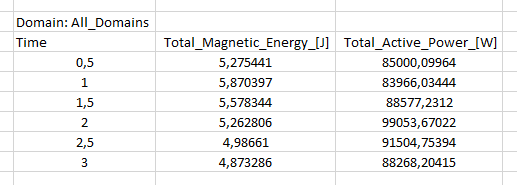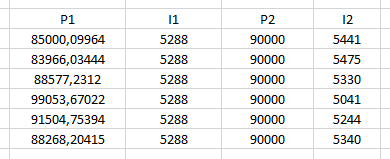# Power controlled simulation

CENOS is a powerful tool that allows you to input *voltage, current , or POWER*** to control your system.

To do so, go to the inductor section of the physics module, there you'll be able to select either voltage, current or power as your control input.The most commonly used induction heating process control is power control, but what happens when you must set it up with voltage or current? When a simulation is built to optimize the heating process, many engineers stumble upon the fact that they instead need to define the current or voltage in the inductor.

## How to find the input current?​

If the current or voltage values in the inductor ar unknown, multiple approaches can be used depending on simulation setup.

### Without B(H) (constant permeability)​

If you do not use a B(H) curve in your simulation, you can easily calculate the current value which corresponds to your desired power value with just one simulation.

info

Run the test calculation without Thermal Analysis, it will run faster and the calculated current value will be correct for simulation with thermal analysis as well!

To do this, you need to follow these steps:

1) Choose one random current value and run 1 time step. You will find the power value corresponding to the current you defined.

$I_1 \to P_1$

2) Without the B(H) curve the power value is proportional to the current. Calculate the necessary current value $I_2$, as the relation between $I_1$ and $P_1$ is known.

$P=UI=I^2R \to \frac{P_1}{P_2}=\frac{I_1^2}{I_2^2}$
$I_2=\sqrt\frac{I_1^2P_2}{P_1}$
example

We have a 3kW power consumption, and we want to calculate the necessary current to put in CENOS.

1) Run one time step with 150 A. From results we see that 150 A current produces 12.45 W power.

2) We use the previously mention equation and calculate the current necessary to produce 3 kW:

$I_2=\sqrt\frac{150^2*3000}{12.45}=2328.45 A$

Once you have calculated the necessary current $I_2$ you can enable thermal analysis, enter the calculated current value and run the simulation with a specified power.

### With B(H)​

If the B(H) curve is used, input current will no longer be proportional to the output power. In such a case you need to take your best guess at what the inductor current could be and run one time step of purely electromagnetic simulation. Take a look at the power value in the results and increase or decrease the current until you reach the desired power consumption.

$I_1 \to P_1$
$I_2 \to P_2$
$I_3 \to P_3$

With this approach you will need to carry out a couple of simulations to find the correct current value. Remember to calculate only 1 time step without thermal analysis!

### μ(T) (heated above Curie temperature)​

If the part is heated above Curie temperature, power controlled simulation is not possible, as the impedance of the system changes around and after Curie point that causes jump in current and voltage values. Because of this, to keep the same power value, there needs to be different current or voltage values before and after the Curie point.

In such a case current or voltage can be defined as table values depending on time. Just run one purely EM calculation with a constant current through all your heating range.

Open the .csv file and take a look at the total calculated power in each time step.As you know the input current, just apply the previously discussed equation to calculate $I_2$ for each time step.Then just copy the new table value into CENOS, and you have found the necessary current table to get a constant power over all heating time!